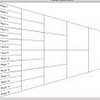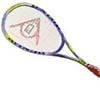#### You may also like### Rain or Shine

Predict future weather using the probability that tomorrow is wet given today is wet and the probability that tomorrow is wet given that today is dry.### Knock-out

Before a knockout tournament with 2^n players I pick two players. What is the probability that they have to play against each other at some point in the tournament?### Squash

If the score is 8-8 do I have more chance of winning if the winner is the first to reach 9 points or the first to reach 10 points?

# How Many Balls?

##### Age 16 to 18Challenge Level

This is another good solution from Alan of Madras College.

Call the number of red balls $r$ and the number of blue balls $b$. The probability of drawing a red ball is $r/(b+r)$ leaving $b$ blue balls and $r-1$ red balls and so the probability of drawing a second red ball is $(r-1)/(b+r-1)$ and the probability of drawing two red balls is $r(r-1)/(b+r)(b+r-1)$. The question tells us that this is five times the probability of drawing two blue balls.

By a similar argument, the probability of drawing two blue balls is $b(b-1)/(b+r)(b+r-1)$.

Also, after one red ball has been drawn the probability of drawing a blue ball is $b/(b+r-1)$ and, considering that you can draw the balls in either order, the probability of a red and a blue ball is $2rb/(b+r)(b+r-1)$. This is six times the probability of drawing two blue balls.

Hence $$\frac{r(r - 1)}{(b + r)(b + r - 1)} = \frac{5b(b - 1)}{(b + r)(b + r - 1)}$$ and we know $(b + r)(b + r - 1) \neq 0$.

\eqalign{ r (r - 1) &= 5b (b - 1) &(1) \\ 2 rb &= 6b (b - 1) &(2)}

From equation (2)

$r = 3b - 3$.

Substituting for $r$ in equation (1) and simplifying the equation gives

$b^2 - 4b + 3 = 0$.

The solutions are $b = 1$ or $b = 3$.

In the case $b = 1$ we have $r = 3b -3 = 0$, but we know $r$ is non zero so $b \neq 1$.

Hence $b = 3$ and $r = 6$, that is there were 6 red balls and 3 blue balls in the bag.